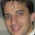## Monday, February 11, 2013

### Label propagation in GraphChi

A few days ago I got a request from Jidong, from the Chinese Renren company to implement label propagation in GraphChi. The algorithm is very simple described here:
Zhu, Xiaojin, and Zoubin Ghahramani. Learning from labeled and unlabeled data with label propagation. Technical Report CMU-CALD-02-107, Carnegie Mellon University, 2002.

The basic idea is that we start with a group of users that we have some information about the categories they are interested in. Following the weights in the social network, we propagate the label probabilities from the user seed node (the ones we have label information about) into the general social network population. After several iterations, the algorithm converges and the output is labels for the unknown nodes.

Here is a pseudo code for the algorithm:

Where Y is the matrix with probabilities for each label, T is the original adjacency graph (where weights are normalized first to one). Clamping means that for the labeled data the weights are fixed to be the input probabilities.

Label propagation is now a part of GraphChi graph analytics toolkit, which includes the following algorithms:
kcores algorithm
subgraph - cut subgraph following X hops around seed nodes / output node degrees
inmemconcomp - in memory connected components

The way to run label propagation is to prepare two input files.
1) The --training=filename file is a file in sparse matrix market format which describes the social graph (an adjacency list of size N x N).
2) Additionally we need to provide a seed file (with the filename: filename.seeds ) which has a sparse matrix of size N x D. D is the number of possible classes categories.
For example, if node 1 is a seed node with 3 categories with probabilities p_1 = 0.3, p_2 = 0.4, p_e = 0.3, we need to add the following inputs:
1 1 0.3
1 2 0.4
1 3 0.3

Here is an example training file for a network of size 5x5 (file name in this example is renren)

%%MatrixMarket matrix coordinate real general
5 5 6
1 2 1
2 1 3
3 4 1
4 5 1
1 5 2
5 2 1

Here is an example seed file (filename is renren.seeds)
%%MatrixMarket matrix coordinate real general
5 4 3
1 1 0.5
1 2 0.3
1 3 0.2

Here is an example run:
bickson@thrust:~/graphchi\$ ./toolkits/graph_analytics/label_propagation --training=renren --D=4 --quiet=1
WARNING:  common.hpp(print_copyright:144): GraphChi Collaborative filtering library is written by Danny Bickson (c). Send any  comments or bug reports to danny.bickson@gmail.com
[training] => [renren]
[D] => 
[quiet] => 

And here is the output of the run:
\$ cat renren_U.mm

%%MatrixMarket matrix array real general

%This file contains LP output matrix U. In each row D probabilities for the Y labels

5 4
5.000000000000e-01
3.000000119209e-01
2.000000029802e-01
0.000000000000e+00
4.999980032444e-01
2.999970912933e-01
2.000028789043e-01
2.033766122622e-06
4.296581745148e-01
6.834248453379e-02
2.388831526041e-01
2.631161808968e-01
4.296608269215e-01
6.834241002798e-02
2.388962060213e-01
2.631005644798e-01
5.000011920929e-01
2.999966442585e-01
1.999970227480e-01
5.159994998394e-06

It is easy to verify the seed node (node 1) probabilities were not changed. But the other nodes have now probabilities which originate in their connections with node 1.

An update: here is a note I got from Soundar Kumara, Prof. in Penn State Univ:

It was nice to see your blog and reference to Label Propagation. Our algorithm (Raghavan, Albert and Kumara, Phys Reviews 2007) was improved further with game theoretic approach.
This is definitely an interesting work. Thanks Soundar!

Next post: Co-EM algorithm in GraphChi

1.Hi Danny,
If the only labeled instance in the example does not have any probability assigned to class 4, how does the output assign weights to class 4, for example, 2.033766122622e-06 on instance 2?

2.As can be seen in the code: https://github.com/GraphChi/graphchi-cpp/blob/master/toolkits/graph_analytics/label_propagation.cpp#L151
non-seed nodes get a random initial label probability and thus you get results for classes which are not observed. You can change this behavior to uniform probability if you lie.

3.Hi Danny, thanks for the tutorial on Label propagation. I have a question about high dimensionality. Does reducing the dimensions of a high dimensional Y matrix using say for example PCA effect the working of the algorithm. I have a high dimensional data set and was wondering do I need to reduce the dimensions before running your tool for good results?

4.Hello, thank you for the implementation of the LP algorithm in Graphchi and for these examples.
In the output, columns and rows seems to be inverted. MatrixMarket array format lists the entries by columns. However, the first 4 entries in renren_U.mm correspond to the first ROW of the matrix according to the header "5 4" which defines 5 rows and 4 columns and correspond to the probability distribution over the 4 classes for node 1. Am I wrong?

1.Hi Ivan,
You are perfectly right, we output by rows and not by columns.

Thanks